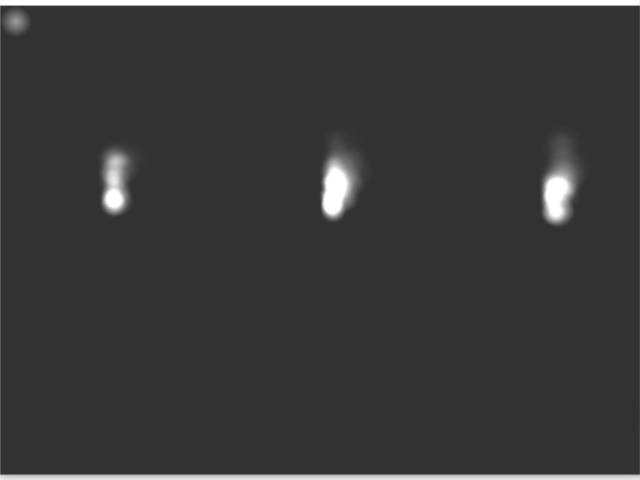-=+=- -=+=- -=+=- -=+=- -=+=- -=+=- -=+=- -=+=- -=+=- -=+=- -=+=- -=+=- -=+=- -=+=- -=+=- -=+=- -=+=- -=+=- -=+=- -=+=- -=+=- -=+=- -=+=- -=+=- -=+=- -=+=- -=+=- -=+=- -=+=- -=+=- (c) WidthPadding Industries 1987 0|376|0 -=+=- -=+=- -=+=- -=+=- -=+=- -=+=- -=+=- -=+=- -=+=- -=+=- -=+=- -=+=- -=+=- -=+=- -=+=- -=+=- -=+=- -=+=- -=+=- -=+=- -=+=- -=+=- -=+=- -=+=- -=+=- -=+=- -=+=- -=+=- -=+=- -=+=-
SoCoder -> Snippet Home -> Graphical Effects

PakzCreated : 16 December 2016
Edited : 16 December 2016
Language : Monkey

### Additive Particle Flames

Flames and additive blendmodeHere the html 5 page :
https://cromdesi.home.xs4all.nl/html5/additiveflames/MonkeyGame.html

I found this youtube video where they sort of explain you how to do it.

Youtube Link

### Comments

Friday, 16 December 2016, 15:40
JayenkaiSo very easy to do, and would love to do more of this sort of thing, but it's a framerate killer on a lot of Android devices (or at least, the way Monkey does it.. I'm assuming there's other methods, but .. *shrugs*)

Having said that, I added Alt-Blend stuff to my new framework a few days ago, but haven't yet stress tested the Android results. Instead, I just got it to auto-disable blend when running on Android. Lazy, but sod it!Friday, 16 December 2016, 18:12
PakzI just tested one with 25 flames on the old ipad mini. Seems to run okay'ish.

The mobile gadgets are getting faster over the years though. The now expensive ipad pro is said to have desktop like speeds.

I wish I could find more sources like that video to learn to code things like fire effects.